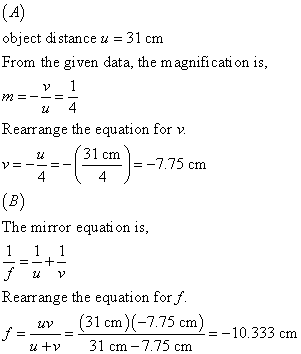# The virtual image produced by a convex mirror is one-quarter the size of the object.

The virtual image produced by a convex mirror is
one-quarterthe size of the object. If the object is 31cm in front of
themirror, what is the image distance?

What is the focal length of
thismirror?

If the object is 31cm in front of
themirror, what is the image distance?
What is the focal length of
thismirror?
What is the focal length of
thismirror? What is the focal length of
thismirror?

object di stance u -31 cm From the given data, the magnificati on is Rearrange the equation for v u–(.31 cm !=-775 cm. The mirror equati on is, Rearrange the equation forf. (31 cm-7.75 cm) -10.33 cm u 31 cm-7.75 cm